Maths-
General
Easy

Question

# The curves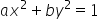and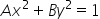intersect orthogonally, then

##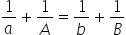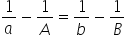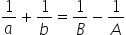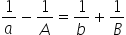Hint:

## The correct answer is:### The curves are as follows:ax2 + by2 = 1            ...(1)Ax2 + By2 = 1          ...(2)        We will take the derivative of equation (1) w.r.t x.2ax + 2by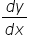=02by= -2ax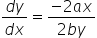This is this slope of first curve. We will call it slope1.In the same way we will take the derivative of equation (2) w.r.t x 2Ax + 2By= 1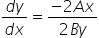We will call it slope2.The two curves are orthogonal. So, the product of their slope is -1.Slope1 × Slope2 = -1We will substitute (3) in (1)We will substitute (4) in (2)Divide (5) by (6)This is the required answer.   .

For such questions, we should be careful while taking the derivative.#### With Turito Foundation.#### Get an Expert Advice From Turito.